# Ground Penetrating Radar Weak Signals Denoising via Semi-soft Threshold Empirical Wavelet Transform

Ground Penetrating Radar Weak Signals Denoising via Semi-soft Threshold Empirical Wavelet Transform
China University of Mining and Technology (Beijing), School of Mechanical Electronic & Information Engineering, Beijing 100083, China

China University of Mining and Technology (Beijing), College of Geophysics and Surveying Engineering, Beijing 100083, China

Corresponding Author Email:
iamqiaoxu@163.com
Page:
207-213
|
DOI:
https://doi.org/10.18280/isi.240213
8 January 2019
|
Accepted:
28 March 2019
|
Published:
10 July 2019
| Citation

OPEN ACCESS

Abstract:

Ground penetrating radar (GPR) weak signals have the characteristics of low signal-to-noise ratio (SNR) and high frequency, which is a major challenge to noise attenuation. In this paper, we propose a GPR denoising approach based on empirical wavelet transform (EWT) combined with semi-soft thresholding. According to the frequency characteristics of signal, a spectrum segmentation strategy is designed. It can adaptively decompose signal and noise into different modes. The mode which contains more valid signals is processed by hard thresholding to reserve amplitude; the other modes which contain useless signals are processed by soft threshold functions to maintain the continuity of the signal. After weak signal denoising by our proposed method, we compared its performance on synthetic and field data using complete ensemble empirical mode decomposition (CEEMD) and synchro squeezed wavelet transform (SWT). The proposed method denoising performance is better than other two methods.

Keywords:

road security, ground penetrating radar, empirical wavelet transform, signal denoising, threshold function

1. Introduction

The underground damages are challenging the road security. These underground damages are usually caused by underground construction, rainfall and so on. Underground damages may cause road slurry, cracking and collapse.

## 1.jpgThe underground damages have become a serious problem a few years ago. Several big voids were found under the road near the construction site of high-rise buildings in Beijing. In addition, lots of underground damages have been reported. For example, Figure 1 shows a road collapse, 10 m long, 5 m wide, and 3 m deep, caused by underground damages.

To prevent road collapse, some people decided to find underground damages. Among various non-destructive tests, a ground penetrating radar (GPR) test was selected because GPR is the most convenient and accurate tool . The GPR test can be carried out while driving on the road, so it is suitable for urban area because it does not need traffic control and can quickly survey large area. Ground penetrating radar signals become weak when investigation depth increases. Ground penetrating radar weak signals have the characteristics of low signal-to-noise ratio and high frequency, which needs to be reduced.

Many methods have been developed for random noise reduction and reflected events recovery. Recently, Time-frequency peak filtering method , singular value decomposition method , local signal-and-noise orthogonalization , and dictionary method , have been exclusively used for GPR data denoising. Because of the high SNR of GPR signals, it is easier to denoise. But these methods are not ideal for GPR weak signals denoising. Some algorithms are applied to GPR weak signals denoising, such as wavelet transform (WT) and empirical mode decomposition (EMD). In order to increase the amplitude of the GPR weak signals, time-reversal-based processing techniques are designed . However, these methods perform poorly in weak reflection events, particularly when the SNR is below a given threshold. The two improved methods of EMD , EEMD  and CEEMD , are effective signal analysis methods. But they have no adequate theoretical basis. Some algorithms are applied to GPR weak signals denoising, like low-rank filtering , synchrosqueezed wavelet transform (SWT)  and Hidden Markov model [12-13], are not suitable for downhole GPR weak signal denoising. Pattern recognition and incremental nonnegative matrix factorization  and neural network  are methods for GPR event detection and SNR enhancement. Whereas, the main work of these methods focus on signal detection instead of SNR enhancement. Multi-resolution analysis algorithms  has good performance for downhole GPR weak signals denoising, but its time complexity is high.

Recently, empirical wavelet transform (EWT) has been proven to be an useful signal analysis method . It applies Littlewood-Paley and Meyer’s wavelets to extracts the amplitude modulated-frequency modulated (AM-FM) components, which are defined as Intrinsic Mode Function (IMF). An equivalent of the Harmonic wavelet, when the basis function is real, is Littlewood–Paley wavelet . Meyer wavelet is a classic wavelet. It has many good properties, for example derivation infinitely, smoothness, attenuates fast . EWT has been applied in many fields. When analyzing the time-frequency characteristics of GPR signals, EWT can provide a high resolution . Meanwhile, it effectively remove the powerline interference and baseline wander in the electrocardiogram signal . In research and application, EWT is a new and effective algorithm.

In this paper, we research on noise attenuation for GPR weak signal via EWT algorithm. EWT effectively decomposes GPR weak signal. Meanwhile, the combination of hard threshold function and soft threshold function, improved threshold function can better preserve signal. As a consequence, underground events become clearer after denoising. This paper is structured as follows. Section II describes the theory of EWT. Section III introduces spectrum segmentation schemes and threshold functions. Section IV presents the results and the comparative analysis. Eventually, Section V concludes this paper.

2. Theory of EWT

EWT is a completely adaptive and data driven method with solid mathematical foundations. The steps for this approach are as follows.

IMF needs to meet the following rules:

1. To acquire signal Fourier spectrum F(ω) , we compute the fast Fourier transform (FFT) of signal f (t).

2. Segment the Fourier spectrum correctly. The set of boundaries is $\Omega=\left\{\omega^{k}\right\}_{k=0,2, \ldots, K}$. Separate the Fourier spectrum [0,π] into K-1 contiguous parts and $\omega^{0}=0$ , $\omega^{K}=\pi$. In Section III, the segmentation method will be introduced in detail.

3. Littlewood-Paley and Meyer wavelets can be used to design low-pass and band-pass filter.

The expression of Littlewood-Paley wavelet is as follows:

$\psi(t)=\frac{\sin (4 \pi t)-\sin (2 \pi t)}{2 \pi t}$         (1)

The expression of Meyer wavelet is as follows:

$\varphi(\omega)=\left\{\begin{array}{ll}{1,} & {|\omega| \leq \frac{2 \pi}{3}} \\ {\cos \left(\frac{\pi}{2} \beta\left(\frac{3|\omega|}{2 \pi}-1\right)\right),} & {\frac{2 \pi}{3} \leq|\omega| \leq \frac{4 \pi}{3}} \\ {0,} & {\text { otherwise }}\end{array}\right.$           (2)

$\psi_{k}(\omega)=\left\{\begin{array}{ll}{e^{j \frac{\omega}{2}} \sin \left(\frac{\pi}{2} \beta\left(\frac{3|\omega|}{2 \pi}-1\right)\right),} & {\frac{2 \pi}{3} \leq|\omega| \leq \frac{4 \pi}{3}} \\ {e^{j \frac{\omega}{2}} \cos \left(\frac{\pi}{2} \beta\left(\frac{3|\omega|}{2 \pi}-1\right)\right),} & {\frac{4 \pi}{3} \leq|\omega| \leq \frac{8 \pi}{3}} \\ {0,} & {\text { otherwise }}\end{array}\right.$          (3)

where, $\varphi(\omega)$  is scaling function and $\psi(\omega)$  is Meyer’s wavelet function.

The empirical scaling function $\psi_{1}(\omega)$  and the empirical wavelets $\psi_{k}(\omega)$  are defined by expressions of equation (4) and (5).

$\varphi_{1}(\omega)=\left\{\begin{array}{ll}{1,} & {|\omega| \leq(1-\gamma) \omega^{1}} \\ {\cos \left(\frac{\pi}{2} \beta\left(\frac{1}{2 \gamma \omega^{1}}-(1-\gamma) \omega^{1}\right)\right),} & {(1-\gamma) \omega^{1} \leq|\omega| \leq(1+\gamma) \omega^{1}} \\ {0,} & {\text { otherwise }}\end{array}\right.$            (4)

$\psi_{k}(\omega)=\left\{\begin{array}{ll}{1,} & {(1+\gamma) \omega^{k} \leq|\omega| \leq(1-\gamma) \omega^{k+1}} \\ {e^{j \frac{\omega}{2}} \sin \left(\frac{\pi}{2} \beta\left(\frac{1}{2 \gamma \omega^{k+1}}-(1-\gamma) \omega^{k+1}\right)\right),} & {(1-\gamma) \omega^{k+1} \leq|\omega| \leq(1+\gamma) \omega^{k+1}} \\ {e^{j \frac{\omega}{2}} \cos \left(\frac{\pi}{2} \beta\left(\frac{1}{2 \gamma \omega^{k}}-(1-\gamma) \omega^{k}\right)\right),} & {(1-\gamma) \omega^{k} \leq|\omega| \leq(1+\gamma) \omega^{k}} \\ {0,} & {\text { otherwise }}\end{array}\right.$        (5)

where, ω is the frequency, ωk is the kth boundary frequency, γ is a parameter that ensures no overlap between the two consecutive transitions, and β(x) is an arbitrary function that satisfies the following conditions such as

$\beta(x)=\left\{\begin{array}{ll}{0,} & {x \leq 0} \\ {1,} & {x \geq 1} \\ {\beta(x)+\beta(1-x)=1,} & {0<x<1}\end{array}\right.$         (6)

Many functions satisfy these conditions, the most commonly used in the literature is

$\beta(x)=x^{4}\left(35-84 x+70 x^{2}-20 x^{3}\right)$         (7)

4. Extract different modes according to the scale function and the empirical wavelet function. The scale coefficients are given by the inner products with the scale function:

$W(1, t)=\langle f, \varphi\rangle=\int f(\tau) \overline{\varphi(\tau-t)} d \tau$            (8)

The detailed coefficients are obtained by the inner product with empirical wavelets:

$W(k, t)=\langle f, \psi\rangle=\int f(\tau) \overline{\psi(\tau-t)} d \tau$         (9)

where, τ is the time point, $\langle\bullet\rangle$ is the inner product, over-bar represents the conjugation of a function and W(k,t) denotes the coefficients for the kth filter bank at the tth time point. The noise coefficients are less than the effective signal coefficients. Then by the mechanism of the scale difference of empirical wavelet coefficients for signal and noise, the empirical wavelet coefficients of noisy are threshold processed.

3. GPR Weak Signal Denoising via EWT

3.1 Segmentation of the Fourier spectrum

The segmentation mechanism of Fourier spectrum is a vital step in separating signals and noise, which provides a theoretical basis for the adaptive analysis of signals. Therefore, even in the case of low SNR, EWT can analyze the signal effectively. There are four segmentation methods in EWT, named localmax, localmaxmin, localmaxminf, and adaptive, respectively. Because adaptive method shows better frequency division ability, it is used in this paper.

The main idea of adaptive method is that starts from an initial set of boundaries and then adapts them as the smallest minimum within a window around considered boundary. The minimum are computed from the original spectrum. Since 0 and π are always used in the first boundary and the last boundary, we need to find extra $k-1$  boundaries. Assuming that initial boundary is set to $a_{k}, k=0,1, \ldots, K$,$a_{k} \in\left[0, \omega_{\max }\right]$ and $a_{0}=0, a_{k}=\omega_{\max }$  ( $\omega_{\max }$  is the maximum frequency of the signal), the window can be calculated by equation (10).

$\left\{\begin{array}{l}{L_{k}=a_{k}-\text { round }\left(\frac{a_{k}-a_{k-1}}{2}\right)} \\ {U_{k}=a_{k}+\text { round }\left(\frac{a_{k+1}-a_{k}}{2}\right)}\end{array}\right.$        (10)

where, round $(\bullet)$ means rounded function. The lower and upper are the lower and upper limits of the window, respectively.

The expression of the boundary is as follows:

$\omega_{k}=\underset{\omega}{\arg \min } F_{l}(\omega), \quad \omega \in\left[L_{k}, U_{k}\right]$        (11)

where, $F_{l}(\omega)$  is minimum value within a window.

To demonstrate the feasibility of adaptive method, we use the sinusoidal wave with SNR equaling to -50 dB to simulate signal. The expression of sinusoidal wave x(t) is as follows:

$x(t)=a_{1} \sin \left(\omega_{1} t\right)+a_{2} \sin \left(\omega_{2} t\right)$        (12)

where, $a_{1}, a_{2}$  are amplitudes $\omega_{1}, \omega_{2}$  are angular frequencies and t is time. The spectrum of the pure signal, noisy and mixed signal are shown in Figure 2, we can see that the dominant frequency of the mixed signal ranges from 0 to 500 MHz. In order to make full use of the spectral characteristics, we set $k=3$ . Boundaries and filtering bank of the adaptive are shown in Figure 3.

## 2.jpgFigure 2. The spectrum of the signal. (a) Pure signal. (b) Noisy. (c) Mixed signal

## 3.pngFigure 3. Boundaries and filtering bank of the adaptive. (a) Boundaries. (b) Filtering bank

## 4.jpgFigure 4. Empirical wavelet coefficients from three subbases. (a) Band1. (b) Band2. (c) Band3

3.2 Threshold functions

Hard threshold function has been widely used, since it is good on keeping signal amplitude. But there are some shortcomings. For example, the reconstructed data will be discontinuous at threshold point; the reconstructed signal is prone to Pseudo-Gibbo phenomenon. A new threshold approach overcomes the disadvantage which is not continuous, but advantage of hard threshold is not preserved.

We assume that $W_{i, t}, i=1,2, \ldots, K, t=1,2, \ldots, N$  is empirical wavelet coefficient matrix of the ith mode. $\widehat{W}_{i, t}$  is coefficient matrix after threshold. $\lambda_{i}$  is threshold which is related to the variance of the noise. $\operatorname{sgn}(\bullet)$  is the sign function. The expressions of hard threshold function, soft threshold function and the improved threshold function are as follows.

Hard threshold function is

$\hat{W}_{i, t}\left\{\begin{array}{ll}{W_{i, t},} & {\left|W_{i, t}\right| \geq \lambda_{i}} \\ {0,} & {\left|W_{i, t}\right|<\lambda_{i}}\end{array}\right.$          (13)

Soft threshold function is

$\hat{W}_{i, t}\left\{\begin{array}{ll}{\operatorname{sgn}\left(W_{i, t}\right)\left(\left|W_{i, t}\right|-\lambda_{i}\right),} & {\left|W_{i, t}\right| \geq \lambda_{i}} \\ {0,} & {\left|W_{i, t}\right|<\lambda_{i}}\end{array}\right.$         (14)

The semi-soft threshold function is

$\hat{W}_{i, t}\left\{\begin{array}{ll}{\mu W_{i, t}+(1-\mu) \operatorname{sgn}\left(W_{i t}\right)\left(\left|W_{i, t}\right|-\lambda_{i}\right),} & {\left|W_{i, t}\right| \geq \lambda_{i}} \\ {0,} & {\left|W_{i, t}\right|<\lambda_{i}}\end{array}\right.$        (15)

where

$\mu=1-\alpha^{\left(|W_{i, t}|-\lambda_{i}\right)^{2}}, 0 \leq \alpha \leq 1,0 \leq \mu \leq 1$          (16)

When $\alpha \rightarrow 0$  is the hard threshold function, $\alpha \rightarrow 1$  is the soft threshold function. In general, $\alpha=0.05$ . Due to subtraction, the coefficients by threshold processed are less than the original coefficients. The amplitude of the denoised signals will be less than the pure signals.

To check the feasibility and validity of our threshold algorithm, we use the four threshold methods to process the synthetic signals. We not only analyze the denoised waveform, but also analyze the denoising results using SNR, peak signal to noise ratio (PSNR), mean square error (MSE) and mean absolute error (MAE). Higher SNR, PSNR and lower MSE, MAE mean better denoising results. The expression are as follows.

$\mathrm{SNR}=101 \mathrm{g}\left(\sum_{t=1}^{N} \frac{F_{i, t}^{2}}{\left(\hat{W}_{i, t}^{2}-F_{i, t}^{2}\right)^{2}}\right)$           (17)

$\operatorname{PSNR}=10 \lg \left(\frac{\left(2^{n}-1\right)^{2}}{\frac{1}{N} \sum_{t=1}^{N}\left(\hat{W}_{i, t}-F_{i, t}\right)^{2}}\right)$         (18)

$\mathrm{MSE}=\sqrt{\frac{\sum_{t=1}^{N}\left(\hat{W}_{i, t}^{2}-F_{i, t}^{2}\right)^{2}}{N}}$          (19)

$\mathrm{MAE}=\frac{\sum_{t=1}^{N}\left|\hat{W}_{i, t}-F_{i, t}\right|}{N}$            (20)

where $F_{i, t}$  is the pure signal, $\widehat{w}_{i, t}$  is the denoised signal, and N is the length of signal.

After signal denoising via CEEMD, SWT, results are shown Figure 5 and Figure 6.

## 5.jpg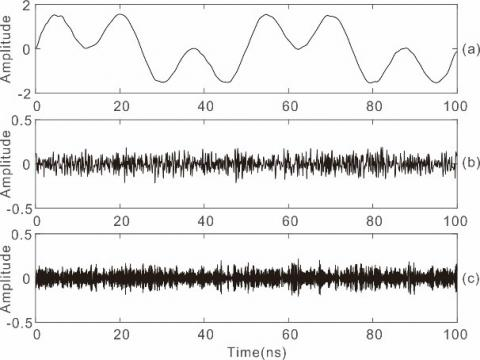Figure 5. IMFs via CEEMD algorithm. (a) IMF5 + IMF4 + IMF3.  (b) IMF2. (c) IMF1

## 6.jpgFigure 6. Mixed signal denoising via SWT. (a) Mixed signal. (b) Denoised signal. (c) Residual signal

We analyze quantitatively the denoising results of the three methods with the help of SNR, PSNR, MSE and MAE.

Table 1. Denoising results of different methods

 Method SNR(dB) PSNR(dB) MSE MAE Mixed signal 19.843 33.987 2.276 0.0815 CEEMD 31.122 39.626 0.621 0.0218 SWT 31.796 39.963 0.575 0.0206 EWT 32.427 40.278 0.534 0.0192

The results show that the proposed algorithm has better performance than CEEMD and SWT methods. Under the proposed algorithm, different threshold functions are compared with the help of SNR, PSNR, MSE and MAE. From Table 2, we can find that semi-soft threshold method obtains the largest SNR and the smallest MSE.

Table 2. Denoising results of different threshold methods

 Method SNR(dB) PSNR(dB) MSE MAE Hard threshold 27.692 34.126 0.913 0.0738 Soft threshold 30.588 38.921 0.701 0.0379 Semi-soft Threshold 31.796 39.963 0.575 0.0206
4. Experiment Results

A synthetic GPR data was used to check the feasibility and validity of EWT algorithm. The comparation of CEEMD, SWT and the proposed algorithms will prove the improvement on previous work. The field GPR data was used to check the practicability and reliability of EWT algorithm.

4.1 Synthetic example

To check the feasibility and validity of EWT algorithm, we use a synthetic GPR data with the dominant frequency of 200 Hz, and the wave velocity is about 1.11´105 km/s. The synthetic GPR data was generated by finite difference-time domain method. The simulative model is as shown in Figure 7a. The sampling frequency is about 10 GHz, and the SNR is -50dB (Figure 7b). The EWT algorithm is compared with CEEMD and SWT algorithm.

## 7.pngFigure 7. GPR synthetic signal. (a) GPR synthetic model. (b) SNR is -50 dB

For A-Scan GPR data (trace number is 180), the results based on three types of methods are shown in Figure 8.

## 8.jpgFigure 8. GPR synthetic signal. SNR is -50 dB

The EWT algorithm is compared with CEEMD and SWT algorithm. For GPR A-Scan signal, the results based on three types of methods are shown in Figure 9. In the result of CEEMD, there is a lot of noise residue near useful signal. In the enlarged part, CEEMD and SWT are all leak signal. In contrast, the proposed algorithm can preserve valid signals better, including peaks and troughs. Meanwhile, a larger amount of random noise is eliminated by using proposed algorithm in smooth regions.

As far as spectrum is concerned, the spectrum of CEEMD almost concentrates in low frequency constituents, and the spectrum of SWT almost concentrates in middle frequency constituents. The spectrum of EWT is closer to the high frequency and low frequency constituents of pure signal.

## 9.pngFigure 9. Results of different denoising method

## 10.jpg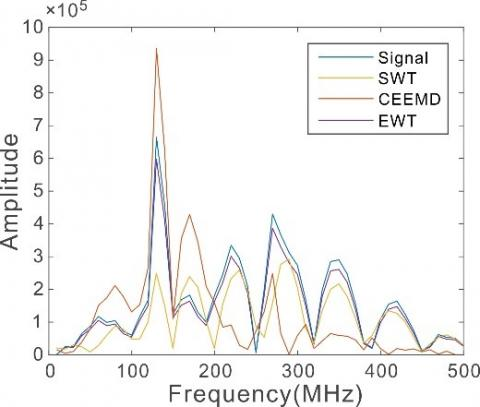Figure 10. The spectrum of different denoising method

## 11.png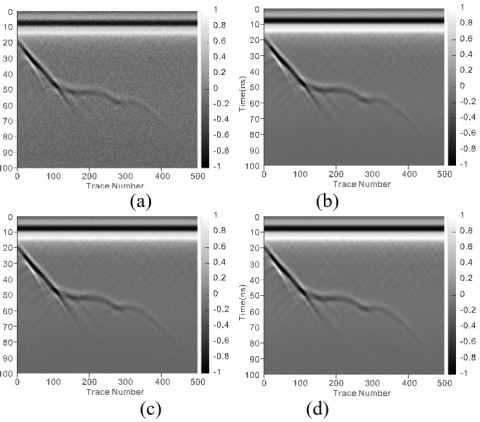Figure 11. Field results of different denoising method. (a) GPR data. (b) Denoising results of SWT. (c) Denoising results of CEEMD. (d) Denoising results of EWT

Table 3. Synthetic GPR data denoising results

 Method SNR(dB) PSNR(dB) MSE MAE Mixed signal -50.000 -0.858 3068.4 216.54 CEEMD -47.966 3.361 2395.0 169.94 SWT -43.315 5.225 1587.7 113.35 EWT -42.027 6.018 1366.7 93.43

## 12.jpg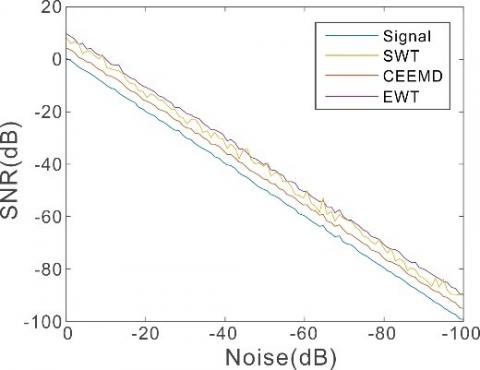Figure 12. SNR results of different denoising method

## 13.jpgFigure 13. MSE results of different denoising method

The SNR , PSNR, MSE and MAE are four objective indexes in GPR signal processing. Table 3 shows the denoising results based on the synthetic GPR data. Comparing with the CEEMD and SWT, the EWT gets a higher SNR and smaller MSE in a variety of situations. We can see that the denoising result weakened when the SNR is below −60dB from Figure 13. Therefore, the SNR of the recorded data is required no less than −60dB for efficient performance of the presented EWT method. When the pure data are unknown for field data denoising tests, the SNR-based evaluation becomes unavailable.

4.2 GPR field data

## 14.jpg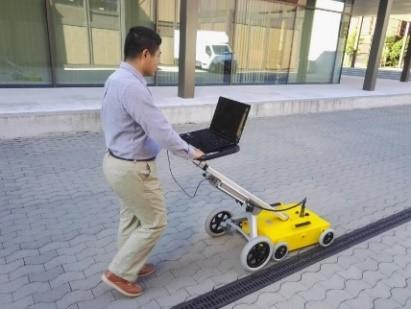Figure 14. 300MHz GPR was used to detect the urban roads

## 15.pngFigure 15. Field results of different denoising method. (a) GPR data. (b) Denoising results of SWT. (c) Denoising results of CEEMD. (d) Denoising results of EWT

In order to prove the practicability and reliability of proposed method, it is necessary to apply this method to actual data. We use the field signals from a GPR with the dominant frequency of 300 MHz (Figure 14). After being enhanced, the GPR weak signals always include a lot of noise (Figure 15a).

In Figure 15, three algorithms are applied to B-Scan GPR signals. It clearly shows that EWT method (Figure 15d) obtains the most satisfactory output, and the events become clearer. At the reflection part, our algorithm keeps the important details of the input signal. The waveform of SWT results (Figure 15b) is distorted. The noise and signal are all reduced in the results of CEEMD (Figure 15c). There are effective signals in the error images of SWT (Figure 15b) and CEEMD (Figure 15c).

## 16.pngFigure 16. The error images of different denoising method. (a) Two regions of GPR data. (b) The error image of E CEEMD. (c) The error image of SWT. (d) The error image of EWT

To display merits of EWT algorithm prominently, three methods are compared in regions A and B. Region A contains a large number of valid signals, and region B contains a lot of noise. Figure 15b, Figure 15c, and Figure 15d are the denoising results of SWT, CEEMD, and EWT methods, respectively. Region B shows that CEEMD denoised signal distortion is greater than SWT. Whereas EWT denoised signal distortion is minimal.  Region A shows that there is a great deal of noise residual. The noise contained in the SWT denoising results is the most, followed by CEEMD, but the noise in the EWT is the least. From the box of residual graph, we can see that CEEMD and SWT algorithms lose the useful signal parts (Figure 16b, Figure 16c). The signal leakage through EWT is less than other two methods (Figure 16d). In suppression noise, the result obtained by proposed method outperforms the results from the CEEMD and SWT. By contrast, we can find that the EWT algorithm can improve the SNR and the continuity of events is better. Therefore, the EWT denoising performance is better than other two methods.

5. Conclusion

This paper presents a new algorithm for GPR weak signal denoising based on EWT. It builds a wavelet filter bank based on Fourier supports detected from the information contained in the processed signal spectrum. In order to increase denoising ability of EWT, it is combined with improved threshold functions to retain effective signals. The experimental results on synthetic and field data show that the CEEMD and SWT algorithm leads to distortion of the reconstructed signal. However, EWT can retain the effective signal while suppressing the noise. Furthermore, we analyze quantitatively the denoising results of the three methods with the help of SNR, PSNR, MSE and MAE. Experiments show that the proposed algorithm have better performance than CEEMD and SWT methods in keeping detail information and improving SNR of reconstruction signal.

Acknowledgment

The Open Foundation of State Key Laboratory for Coal Resources and Safety Mining (SKLCRSM19KFA07). China Railway Corporation Research Project (2017G002-Q). The Science and Technology Project in Guizhou (GZ 3020). Anhui Hengyuan Coal & Electric Corporation Technological Development Project. The Yue Qi Distinguished Scholar Project.

References

 Elkarmoty, M., Colla, C., Gabrielli, E., Bondua, S., Bruno, R. (2017). Deterministic three-dimensional rock mass fracture modeling from geo-radar survey: A case study in a sandstone quarry in Italy. Environmental and Engineering Geoscience, 23(4): 313-330. https://doi.org/10.2113/EEG-1900

 Liu, C., Yang, B.J., Lu, Q., Feng, X., Liu, Y., Wang, D. (2012). Fundamental geophysical progress on Heilongjiang plate tectonics. Journal of Jilin University (Earth Science Edition), 42(5): 1497-1505.

 Sharma, P., Kumar, B., Singh, D., Gaba, S.P. (2017). Critical analysis of background subtraction techniques on real GPR data. Defence Science Journal, 67(5): 559-571. https://doi.org/10.14429/dsj.67.10048

 Chang, H.C., Huang, Y., Hsu, K.T., Lee, K.F., Chiang, C.H. (2016). In depressing the noise effects shown in GPR images by employing the non-local approach. 16th International Conference of Ground Penetrating Radar, GPR, 2016. https://doi.org/10.1109/ICGPR.2016.7572650

 Ozkaya, U., Seyfi, L. (2018). Deep dictionary learning application in GPR B-scan images. Signal, Image and Video Processing, 12(8): 1567-1575. https://doi.org/10.1007/s11760-018-1313-x

 Santos, V.R.N., Teixeira, F.L. (2017). Application of time-reversal-based processing techniques to enhance detection of GPR targets. Journal of Applied Geophysics, 146: 80-94. https://doi.org/10.1016/j.jappgeo.2017.09.004

 Feng, D.S., Dai, Q.W., Yu, K. (2012). GPR signal processing under low SNR based on empirical mode decomposition. Journal of Central South University (Science and Technology), 43(2): 596-604.

 Gan, L., Zhou, L., Liu, S.M. (2014). In A de-noising method for GPR signal based on EEMD. 2014 International Conference on Manufacturing Technology and Electronics Applications, ICMTEA 2014 3909-3913. https://doi.org/10.4028/www.scientific.net/AMM.687-691.3909

 Li, J., Liu, C., Zeng, Z., Chen, L. (2015). GPR signal denoising and target extraction with the CEEMD method. IEEE Geoscience and Remote Sensing Letters, 12(8): 1615-1619. https://doi.org/10.1109/LGRS.2015.2415736

 Liu, Y., Wang, Q., Zhao, B., Liu, X.J. (2016), In Compartmental low-rank filtering of radio-echo sounding data. 16th International Conference of Ground Penetrating Radar, GPR, 2016. https://doi.org/10.1109/ICGPR.2016.7572600

 Xu, J., Ren, Q., Shen, Z. (2016). 1893. Ground-penetrating radar time-frequency analysis method based on synchro squeezing wavelet transformation. Journal of Vibroengineering, 18(1): 315-323.

 Yuksel, S.E., Bolton, J., Gader, P. (2015). Multiple-instance hidden Markov models with applications to landmine detection. IEEE Transactions on Geoscience and Remote Sensing, 53(12): 6766-6775. https://doi.org/10.1109/TGRS.2015.2447576

 Carrer, L., Bruzzone, L. (2017). Automatic Enhancement and detection of layering in radar sounder data based on a local scale hidden Markov model and the Viterbi algorithm. IEEE Transactions on Geoscience and Remote Sensing, 55(2): 962-977. https://doi.org/10.1109/TGRS.2016.2616949

 Kumlu, D., Erer, I. (2019). Detection of buried objects in ground penetrating radar data using incremental nonnegative matrix factorization. Remote Sensing Letters, 10(7): 649-658.

 Harkat, H., Ruano, A.E., Ruano, M.G., Bennani, S.D. (2019). GPR target detection using a neural network classifier designed by a multi-objective genetic algorithm. Applied Soft Computing Journal, 79: 310-325.

 Li, Z., Wen, J., Xiao, Z., Wang, M. (2019). Comparative evaluation of denoising of tree radar B-scan images using multi-resolution analysis algorithms. International Journal of Circuits, Systems and Signal Processing, 13: 205-212.

 Gilles, J., Tran, G., Osher, S. (2014). 2D empirical transforms. Wavelets, ridgelets, and curvelets revisited. SIAM Journal on Imaging Sciences, 7(1): 157-186. https://doi.org/10.1137/130923774

 Tang, B.P., Li, F., Chen, R.X. (2011). Fault diagnosis based on Littlewood-Paley wavelet support vector machine. Journal of Vibration and Shock, 30(1): 128-131. https://doi.org/10.1631/jzus.A1000141

 Wu, M.T. (2019). Wavelet transform based on Meyer algorithm for image edge and blocking artifact reduction. Information Sciences, 474: 125-135. https://doi.org/10.1016/j.ins.2018.09.058

 Wang, Y., Chen, W., Sun, C., Sun, Q., Huang, H. (2017). Early weak fault diagnosis of gearboxes based on energy aggregation and EWT. China Mechanical Engineering, 28(12): 1484-1490.

 Singh, O., Sunkaria, R.K. (2015). Powerline interference reduction in ECG signals using empirical wavelet transform and adaptive filtering. Journal of Medical Engineering and Technology, 39(1): 60-68. https://doi.org/10.3109/03091902.2014.979954